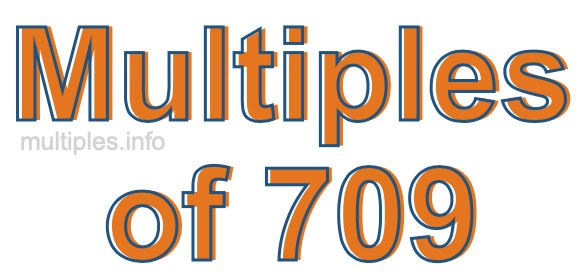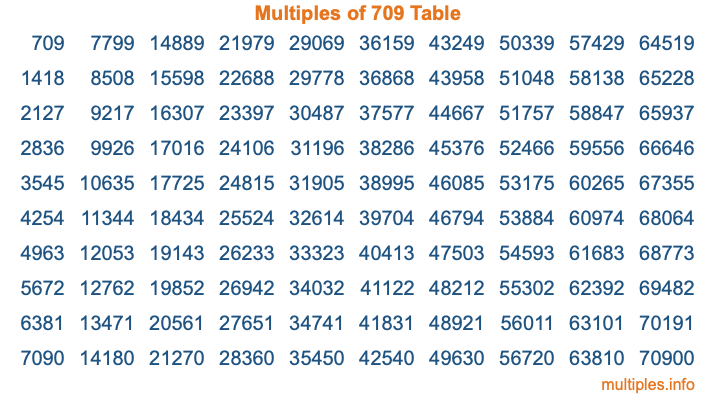Multiples of 709Welcome to the Multiples of 709 page. Here we will first teach you everything you will ever need to know about the multiples of 709, and then give you a study guide summary of everything we taught you to make sure you remember it all. Use this page to look up facts and learn information about the multiples of 709. This page will make you a multiples of seven hundred nine expert!

Definition of Multiples of 709
Multiples of 709 are all the numbers that when divided by 709 equal an integer. Each of the multiples of 709 are called a multiple. A multiple of 709 is created by multiplying 709 by an integer.

Therefore, to create a list of multiples of 709, you start with 1 multiplied by 709, then 2 multiplied by 709, then 3 multiplied by 709, and so on for as long as you want. Thus, the list of the first five multiples of 709 is 709, 1418, 2127, 2836, and 3545. To see a larger list of multiples of 709, see the printable image of Multiples of 709 further down on this page. We also have a category where you can choose any nth multiple of 709.

Multiples of 709 Checker
The Multiples of 709 Checker below checks to see if any number of your choice is a multiple of 709. In other words, it checks to see if there is any number (integer) that when multiplied by 709 will equal your number. To do that, we divide your number by 709. If the the quotient is an integer, then your number is a multiple of 709.

Is  a multiple of 709?

Least Common Multiple of 709 and ...
A Least Common Multiple (LCM) is the lowest multiple that two or more numbers have in common. This is also called the smallest common multiple or lowest common multiple and is useful to know when you are adding our subtracting fractions. Enter one or more numbers below (709 is already entered) to find the LCM.

Check out our LCM Calculator if you need more details about the Least Common Multiple or if you need the LCM for different numbers for adding and subtraction fractions.

nth Multiple of 709
As we stated above, 709 is the first multiple of 709, 1418 is the second multiple of 709, 2127 is the third multiple of 709, and so on. Enter a number below to find the nth multiple of 709.

th multiple of 709

Multiples of 709 vs Factors of 709
709 is a multiple of 709 and a factor of 709, but that is where the similarities end. All postive multiples of 709 are 709 or greater than 709. All positive factors of 709 are 709 or less than 709.

Below is the beginning list of multiples of 709 and the factors of 709 so you can compare:

Multiples of 709: 709, 1418, 2127, 2836, 3545, etc.

Factors of 709: 1, 709

As you can see, the multiples of 709 are all the numbers that you can divide by 709 to get a whole number. The factors of 709, on the other hand, are all the whole numbers that you can multiply by another whole number to get 709.

It's also interesting to note that if a number (x) is a factor of 709, then 709 will also be a multiple of that number (x).

Multiples of 709 vs Divisors of 709
The divisors of 709 are all the integers that 709 can be divided by evenly. Below is a list of the divisors of 709.

Divisors of 709: 1, 709

The interesting thing to note here is that if you take any multiple of 709 and divide it by a divisor of 709, you will see that the quotient is an integer.

Multiples of 709 Table
Below is an image of the first 100 multiples of 709 in a table. The table is in chronological order, column by column. The first column has the first ten multiples of 709, the second column has the next ten multiples of 709, and so on.The Multiples of 709 Table is also referred to as the 709 Times Table or Times Table of 709. You are welcome to print out our table for your studies.

Negative Multiples of 709
Although not often discussed or needed in math, it is worth mentioning that you can make a list of negative multiples of 709 by multiplying 709 by -1, then by -2, then by -3, and so on, to get the following list of negative multiples of 709:

-709, -1418, -2127, -2836, -3545, etc.

Multiples of 709 Summary
Below is a summary of important Multiples of 709 facts that we have discussed on this page. To retain the knowledge on this page, we recommend that you read through the summary and explain to yourself or a study partner why they hold true.

There are an infinite number of multiples of 709.

A multiple of 709 divided by 709 will equal a whole number.

709 divided by a factor of 709 equals a divisor of 709.

The nth multiple of 709 is n times 709.

The largest factor of 709 is equal to the first positive multiple of 709.

709 is a multiple of every factor of 709.

709 is a multiple of 709.

A multiple of 709 divided by a divisor of 709 equals an integer.

709 divided by a divisor of 709 equals a factor of 709.

Any integer times 709 will equal a multiple of 709.

Multiples of a Number
Here you can get the multiples of another number, all with the same attention to detail as we did for multiples of 709 on this page.

Multiples of
Multiples of 710
Did you find our page about multiples of seven hundred nine educational? Do you want more knowledge? Check out the multiples of the next number on our list!

Copyright  |   Privacy Policy  |   Disclaimer  |   Contact